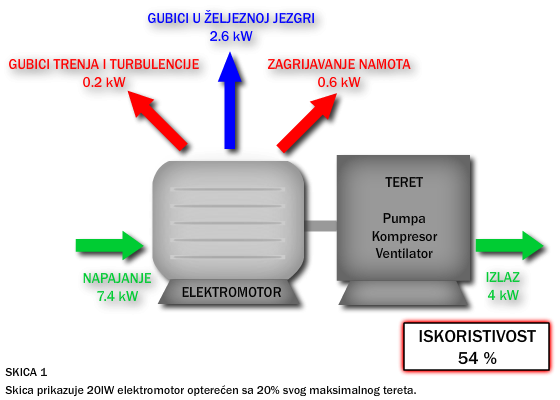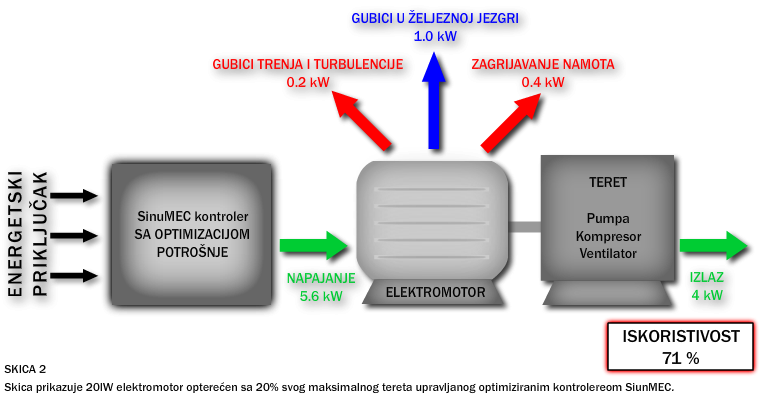### Uvodni primjer:

If we consider the 20kW electric motor to the output shaft has a small load (20% of its maximum) can be noted that the heating coil consumes 0.6kWh and the losses in the iron core are additional 2.6kWh. Overall efficiency of electric motors is 54% (see first illustration).
If using the optimized method of starting and operation of electric motors, can be saved 1.8kW / h of energy.The losses in the winding is reduced to 0.4kWh and the losses in the iron core to 1 kWh.
The system efficiency is 71% (as shown in the figure below)With a saving of 1.8kWh, one can easily calculate the annual savings.

Primjer:
Counting the cost of electricity per kWh L 0.5 can easily calculate the annual savings in cash.

###### 6.289,00 kn

The above savings is just an example, but a good showing saving money and energy.

### The advantages of using energy-efficient motor-controller

It is known that if the induction motor connected to the supply voltage (V 3x400), and if the load on its axis is reduced, that reduces the utilization factor (cos phi) engine! Inductive current component (magnetizing current) remains constant and the reduced load on the motor.
Magnetizing current accounts for 30% of total electricity, so if it is reduced, reducing the losses in the windings, iron losses, dissipation of magnetic flux ... Reducing losses engine is to reduce the input voltage and improve the utilization factor of the motor.

Used engine optimization:
- reduced energy consumption by 30%
- reduced losses in the incoming lines
- smanjuje se struja pokretanja motora
- the reduced voltage and current, motor and mechanical components will have a longer service life
- reducing the inductive component of the current and can be installed less compensatory batteries

Financial benefits:
- reduce maintenance costs is 800 per annum
- reduces wear of mechanical components for the 150 per annum
- the reduced voltage and current, engine life increases 20%, and it brings a saving of 2000 per annum
(will have to be changed or the engine prematati 15 years)
- optimized operation of the motor reduces energy losses from 21% to 30%, and this brings 4000-9000 Kuna annual savings

If we consider all the components, it can be concluded that with the benefits of using energy-efficient motor controller great.

It is important to note that the profitable use frequency controller for applications where constant speed, since the frequency controller is not energy efficient.